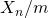## Random Numbers

Ever wonder how we can write simulations simulating real world situations? Consider a simulation of molecules of hydrogen gas in a cylinder. To start the simulation there has to be some initial random movement of the molecules, otherwise there will be no movement at all. Also to determine realistic trajectories we need to introduce some random noise to simulate the effects of external forces to the cylinder. The question is simple. How do we do something randomly, on a computer? We need random numbers. As easy as it may seem it is not at all easy to generate random numbers. Computers are not human beings, they don’t do random. Also modern computers are not analog, they cannot simply produce random digits out of nowhere. Initial computers use random noise in their resistor circuits to generate random numbers. But as we moved to the digital computer systems this problem became bigger and bigger. There are multiple algorithms to find random number we need one which is fast and requires least resources.
A wonderful comic strip by xkcd shows an elegant solution.

Sadly this solution is flawed for obvious reasons.  In this post I will try to explore some authentic ways of generating random numbers.

## What are Random Numbers?

As the name suggests random numbers are random in nature. It mean that given a sufficiently huge list of such numbers there is no real pattern of their occurrence. Also given the nth random number one should not be able to predict the mth random number where m > n.  Furthermore the list should be consistent with some kind of distribution. In general unless specified the distribution is linear which means that if the upper and the lower bound of the numbers are known and there are only N possible random numbers possible between the bounds(this can be  attributed to the limitation of the precision of a machine), then probability of each number occurring in the list is.

## Do we really need Random Numbers?

The answer is No. For most practical purposes it is sufficient to find a pseudo-random numbers or quasi-random numbers. These number sequences are sometime also known as “apparently random sequence” since they seem random. In this post we see how we can generate seemingly random or quasi-random numbers.

## Von Nuemann's Method

This method is inherently flawed but was developed for early machines, with limited computational capabilities. Suppose we have a 3 digit number and we need to find the next 3 digit number which is random.
Consider a starting number as 112. We then square this number and take the middle 3 digits.

This means the next number is 254.  If we keep doing it over and over again, we will build ourselves a list of pseudo-random/quasi-random numbers.

The problem with this approach is that this sequence is often affected by the kind of digits that are generated and digits like zeros and ones often stop the progression. This method is therefore not practical.

## The Linear Congruential Method

This method is also known as Lehmer’s method after D.H. Lehmer. In this method we choose 4 magic numbers. m, a, c, X. Here 0 and m are the lower and upper bounds respectively for a, c, X and m > 0. Once we have these four numbers we define the sequence as

If you choose a = c = X = 3 and m = 10 we have the following progression.

3, 2, 9, 0, 3, 2, 9, 0 …

This means that we have a sequence but the period of reoccurrence of repeated pattern is very small.  For increasing the length of we can simply choose a really big m. We will discuss this a little later. Next we will try to look at some trivial values of a and c. c = 0 is an interesting sequence but it will give you a seemingly random sequence for a big value of m. a= 0 and a= 1 however cannot be used since the progression will no longer be random.  This means for all practical purposes a >= 2.

Next we will try to find the (n+k)th term of the sequence once the nth term is given.  Considering
b= a-1 ( b >= 1 since a >= 2) we have

Then according to above equation

## How to choose the modulo?

In general if we choose the modulus as 2w where w= word size of the machine we are good.  Since this means two things, the range of the number is sufficiently big to suite our practical needs and it is easy to calculate the modulus since the result of the modulo is usually the lower half of the result during multiplication.

## How to implement rand() function?

The random number generator in C/C++ (or any other language for that matter) takes a seed as an input and gives you the a random number between 0 and 1.  This can be achieved by using the random seed to get the value of a, c, X0 and then using the above formula to generate X1. Since ‘m’ is fixed for us the output will always be less than m, we can always returnwhich will lie between 0 and 1.

## Analysis of distribution

To analyze how the random numbers generated by this method are distributed I made a small implementation and generated 1000 numbers between 0-99. The distribution came out to be "essentially linear".

## Conclusion

To conclude this method is easy to implement and generating a random number will be a constant time operation, and essentially amazing for all practical purposes.